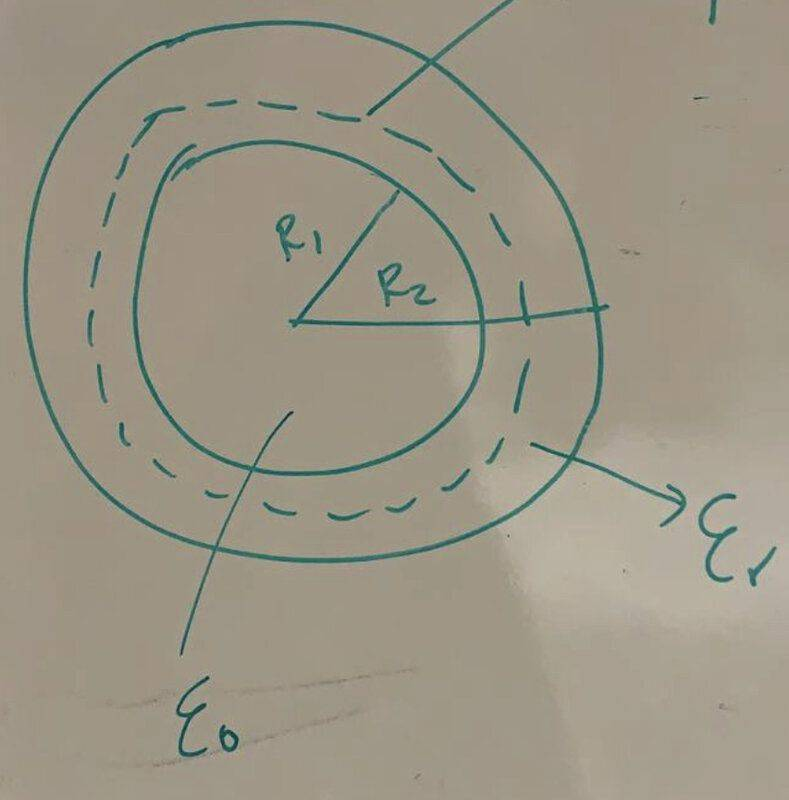# Capacitance of a spherical capacitor

Homework Statement:
A spherical capacitor is formed by two thin conductive layers, spherical and concentric, of radius $R_1$ and $R_2>R_1$, between which we have placed a dielectric material of relative permittivity $\varepsilon_r$. Knowing that the inner layer has an $Q$ charge, idetermines the capacity of the capacitor and the total energy stored.
Relevant Equations:
Gauss Law
When I try to do Gauss, the permeability is not always that of the free space, but it varies: up to a certain radius it is that of the void and then it is the relative one. How can I relate them? I'm trying to calculate the capacity of a spherical capacitor.

The scheme looks like this: inside I have the free space and between the plates of the capacitor I have the dielectric material.The broken lines represent the Gaussian surface.

hutchphd
Homework Helper
What is the result if it were space ( ε0) between the spherical shells?

kuruman
Homework Helper
Gold Member
The vacuum doesn't matter because it contains no charge. The capacitor consists of two conducting plates with the space between them filled completely with the dielectric. Use a Gaussian surface completely inside the dielectric. Or you can find the capacitance with no dielectric between the shells and then multiply it by the dielectric constant.

I think I have the solution. Is that right?
$$\left. \phi =\oint \vec{E}\cdot d\vec{S}=\oint E\cdot dS\cdot \underbrace{\cos 0}_1=E\oint dS=E\cdot S \atop \phi =\dfrac{Q_{enc}}{\varepsilon_0 \varepsilon_r}=\dfrac{Q}{\varepsilon_0 \varepsilon_r}=\dfrac{\sigma \cdot S}{\varepsilon_0 \varepsilon_r} \right\} E\cdot S=\dfrac{\sigma S}{\varepsilon_0 \varepsilon_r}\rightarrow E=\dfrac{\sigma}{\varepsilon_0 \varepsilon_r}=\dfrac{Q}{4\pi R^2 \varepsilon_0}$$
$$C=\dfrac{Q}{V_2-V_1}$$
$$V_2-V_1=-\int_{R_1}^{R_2} \vec{E}\cdot d\vec{l}=-\int_{R_1}^{R_2}E\cdot \overbrace{dl\cdot \cos \theta}^{dR}=-\int_{R_1}^{R_2}\dfrac{Q}{4\pi R^2 \varepsilon_0 \varepsilon_r}dR=\dfrac{Q}{4\pi \varepsilon_0 \varepsilon_r}-\int_{R_1}^{R_2} \dfrac{1}{R^2}dR$$
because $Q$ is constant as it has been transferred to us by an external field/generator. Therefore, it is invariant. $V$ varies due to distance. Then
$$V_2-V_1=\dfrac{Q}{4\pi \varepsilon_0 \varepsilon_r}\left( -\dfrac{1}{R_2}+\dfrac{1}{R_1}\right) \rightarrow C=\dfrac{4\pi \varepsilon_0}{\left( -\frac{1}{R_2}+\frac{1}{R_1}\right)}=\boxed{4\pi \varepsilon_0 \varepsilon_r\dfrac{R_2R_1}{R_2-R_1}}$$

kuruman
•